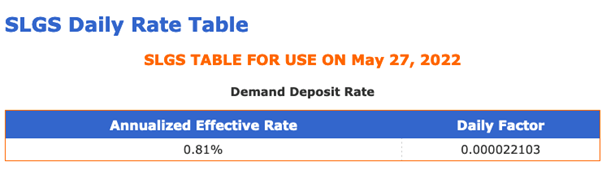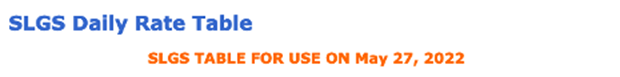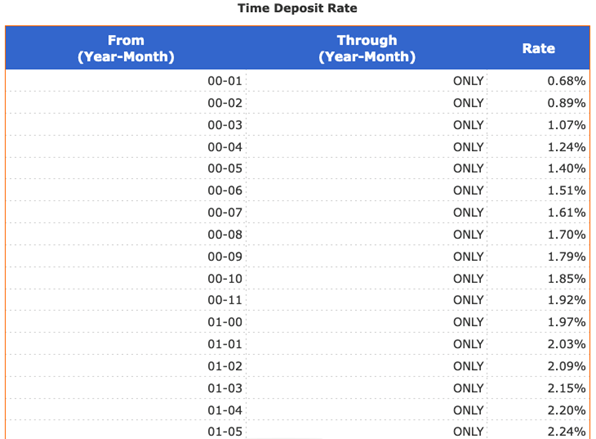SLGS

# How to Read the SLGS Daily Rate Table

Treasury calculates the SLGS Daily Rate table every federal business day and posts it by 10:00 a.m. Eastern U.S. time.

The table includes interest rates for securities with a maturity ranging from one month to 40 years.

It also includes both the rate for Demand Deposit securities and Time Deposit securities.

On this page, we use part of the SLGS Daily Rate table for May 27, 2022, as the example to explain how to read the table.

## Interpreting the Demand Deposit Rate

The Demand Deposit rate is the same for all outstanding Demand Deposit securities.

We reset the rate every federal business day.

We base the interest rate for Demand Deposit securities on an adjustment of the average yield in the most recent auction of 13-week Treasury bills.

As you can see, on May 27, 2022, the annualized effective rate was 0.81% and the daily factor was 0.000022103.To see the current daily rate for Demand Deposit securities, go to the SLGS Daily Rate Table.

## Interpreting the Time Deposit Rate

For Time Deposit securities, the interest rate earned is one basis point below the current Treasury borrowing rate for a security of comparable maturity.

To find your maximum allowable rate for Time Deposit securities, you must know the security's life (that is, how long between the time it is issued and the time it matures).

In the Daily Rate table, the columns headed From and Through refer to the security’s life.

For example, if you had a security that was issued on May 27, 2022, and matured on May 27, 2023, the security's life is exactly one year.

If the security’s life is more than one time increment, but not quite the next increment, use the shorter term. For example, if you will hold the security for 65 days, use the 00-02 (two month) rate, not the 00-03 (three month) rate.The table continues for many rows.

Using this partial table as an example, if you had been looking on May 27, 2022:

• For the security whose life is exactly one year, you would have used the 01-00 row (one year, zero months) and seen that the maximum interest rate allowable on that day was 1.97%
• For the 65 day security, you would have used the 00-02 row and seen that the maximum interest rate allowable on that day was 0.89%.

You may choose any interest rate from 0% to the maximum allowable for your security. You do not have to accept the maximum allowable rate. But you cannot choose an interest rate higher than the maximum allowable rate.

To see the current daily rate for Time Deposit securities, go to the SLGS Daily Rate Table.